•• # Breadth First Search Algorithm

A breadth first search traversal method, visits all the successors of a visited node before visiting any successor of any of its child nodes. This is a contradiction to depth first traversal method; which visits the successor of a visited node before visiting any of its brothers, i.e., children of the same parent. A depth first traversal method tends to create very long, narrow trees; whereas breadth first traversal method tends to create very wide, short trees.

Given an input graph G = (V, E) and source vertex s, from where to begin. BFS systematically explores the edges of G to discover every vertex that is reachable from s. It produces a breadth first tree with root s that contains all such vertices that are reachable from s. For every vertex v reachable from s, the path in the breadth first tree from s to v corresponds to a shortest path. During the execution of the algorithm, each node n of G will be one of the three states, called the status of n as follows:

• Status = 1 (ready state) : the initial state of a node n.
• Status = 2 (waiting state) : the node n is on the queue or stack waiting to be processed.
• Status = 3 (processed state) : the node has been processed.

Undirected GraphAdjacency list for above undirected graph.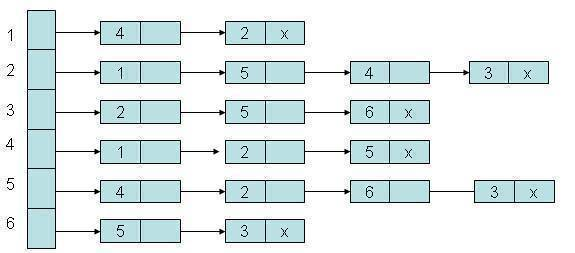Algorithm:

Step 1: Initialize all nodes to ready state (status = 1)

Step 2: Put the starting node in queue and change its status to the waiting state (status = 2)

Step 3: Repeat step 4 and 5 until queue is empty

Step 4: Remove the front node n of queue. Process n and change the status of n to the processed state (status = 3)

Step 5: Add to the rear of the queue all the neighbors of n that are in ready state (status = 1), and change their status to the waiting state (status = 2). [End of the step 3 loop]

Step 6: Exit

Step 1: Initially add 2 to the queue.F=0

R=0

Step 2: Remove the front element 2 from queue by setting front = front +1 add to the queue the neighbors of 2.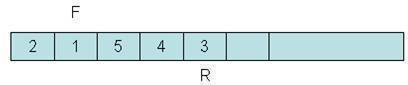F=1

R=4

Step 3: Remove the front element 1 from queue by setting front = front +1 add to the queue the neighbors of 1.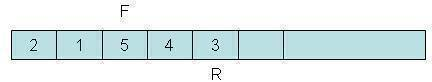F=2

R=4

Step 4: Remove the front element 5 from queue by setting front = front +1 add to the queue the neighbors of 5.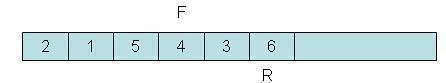F=3

R=5

Step 5: Remove the front element 4 from queue by setting front = front +1 add to the queue the neighbors of 4.F=4

R=5

Step 6: Remove the front element 6 from queue by setting front = front +1 add to the queue the neighbors of 6F=5

R=5

Program illustrating Breadth First Search Algorithm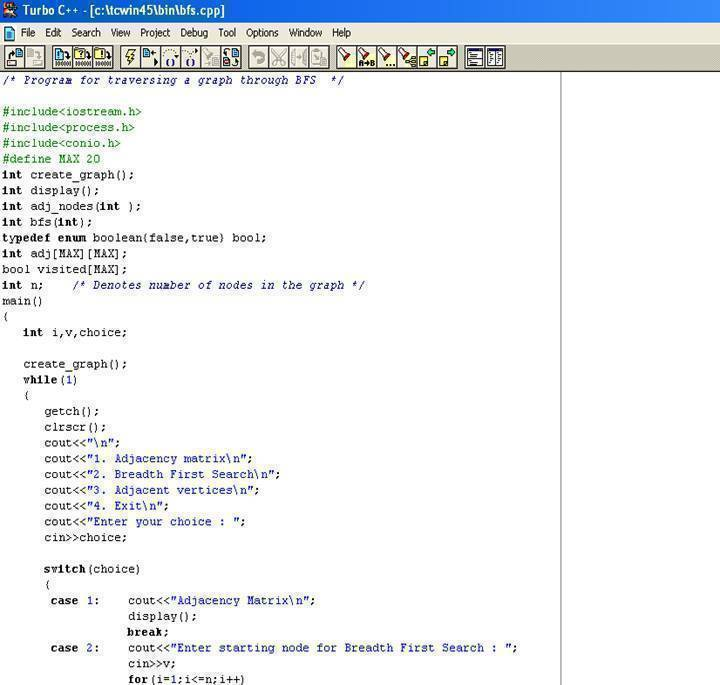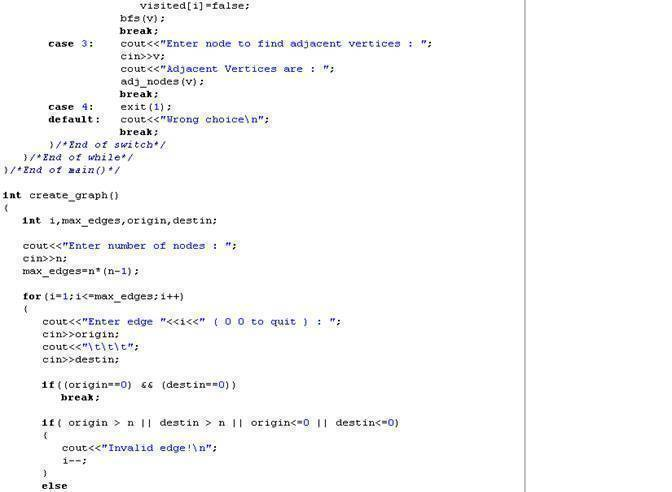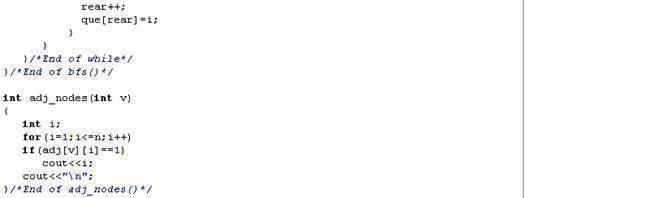Output: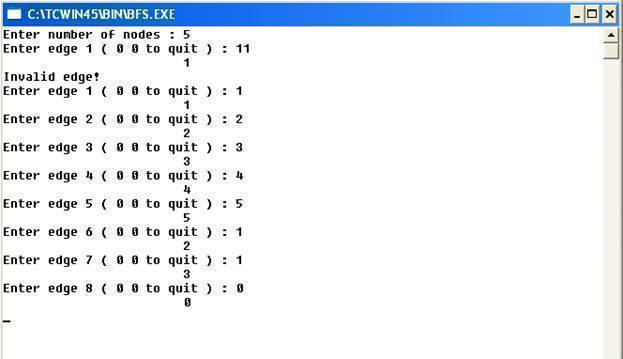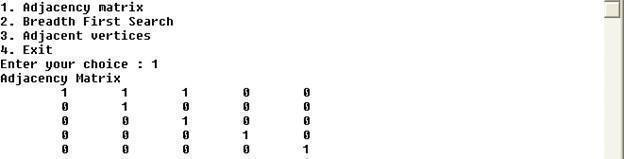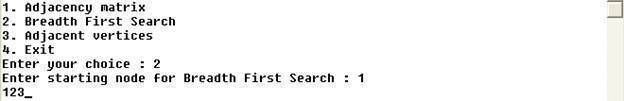1. #### tanvir

24 February, 2012 at 4:12 pm

need description

Reply
175 queries in 0.751 seconds.